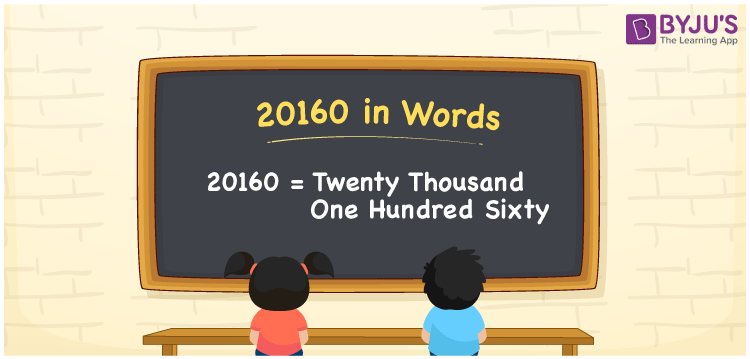# 20160 in Words

20160 in words is twenty thousand one hundred sixty. For example, if you saved Rs. 20160 in a month, you can write, “I have saved Rs. twenty thousand one hundred sixty in a month”. In general, the number name of any number can be written using the ones, tens, hundreds, and thousands place of a number. Thus, the place value chart is useful for writing the number 20160 in words.

 20160 in words Twenty thousand one hundred sixty Twenty thousand one hundred sixty in Numbers 20160

## 20160 in English Words## How to Write 20160 in Words?

We can convert 20160 to words using a place value chart. This can be done as follows. The number 20160 has 5 digits, so let’s make a chart that shows the place value up to 5 digits.

 Ten thousands Thousands Hundreds Tens Ones 2 0 1 6 0

Thus, we can write the expanded form as:

2 × Ten thousand + 0 × Thousand + 1 × Hundred + 6 × Ten + 0 × One

= 2 × 10000 + 0 × 1000 + 1 × 100 + 6 × 10 + 0 × 1

= 20160

= Twenty thousand one hundred sixty

20160 is the natural number that is succeeded by 20159 and preceded by 20161.

20160 in words – Twenty thousand one hundred sixty

Is 20160 an odd number? – No.

Is 20160 an even number? – Yes

Is 20160 a perfect square number? – No

Is 20160 a perfect cube number? – No

Is 20160 a prime number? – No

Is 20160 a composite number? – Yes

## Frequently Asked Questions on 20160 in Words

Q1

### How to write 20160 in words?

20160 in English is written as “twenty thousand one hundred sixty”.
Q2

### Is the number 20160 an odd number?

No, the number 20160 is not an odd number.
Q3

### Is 20160 a composite number?

Yes, 20160 is a composite number.